# Area of Rectangle

Go back to  'Area'

 1 Introduction to Area 2 Definition of Area of a Rectangle 3 Area of a Rectangle Formula 4 Important Notes on Area of a Rectangle 5 Solved Examples on Area of a Rectangle 6 Challenging Questions on Area of a Rectangle 7 Practice Questions on Area of a Rectangle 8 Maths Olympiad Sample Papers 9 Frequently Asked Questions (FAQs)

We at Cuemath believe that Math is a life skill. Our Math Experts focus on the “Why” behind the “What.” Students can explore from a huge range of interactive worksheets, visuals, simulations, practice tests, and more to understand a concept in depth.

Book a FREE trial class today! and experience Cuemath’s LIVE Online Class with your child.

## Introduction to Area

The area of any shape is the number of unit squares that can fit into it.

What is a unit square?

A unit square is a square of side $$1$$ unit.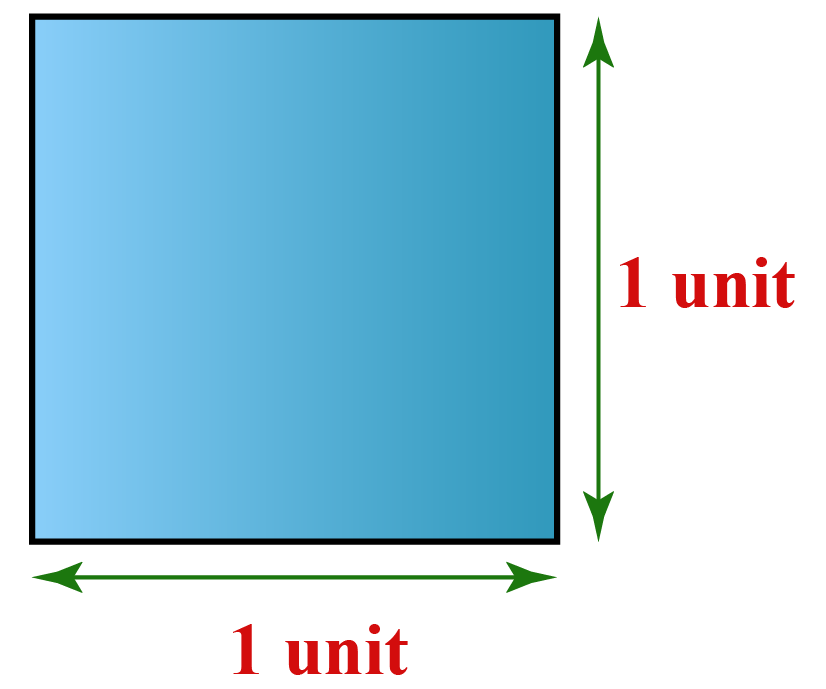Here "unit" can refer to any unit such as centimeter, meter, kilometer, etc.

Let us do a small activity to understand about area using unit squares.

Let's find the area of some shapes by placing them on a grid paper of unit squares and counting the number of unit squares inside the shape.

The area of any shape is measured in square units such as $$\text{cm}^2$$, $$\text{m}^2$$, $$\text{km}^2$$ etc depending on the given unit.

If no unit is given, the unit of area is considered to be "square units".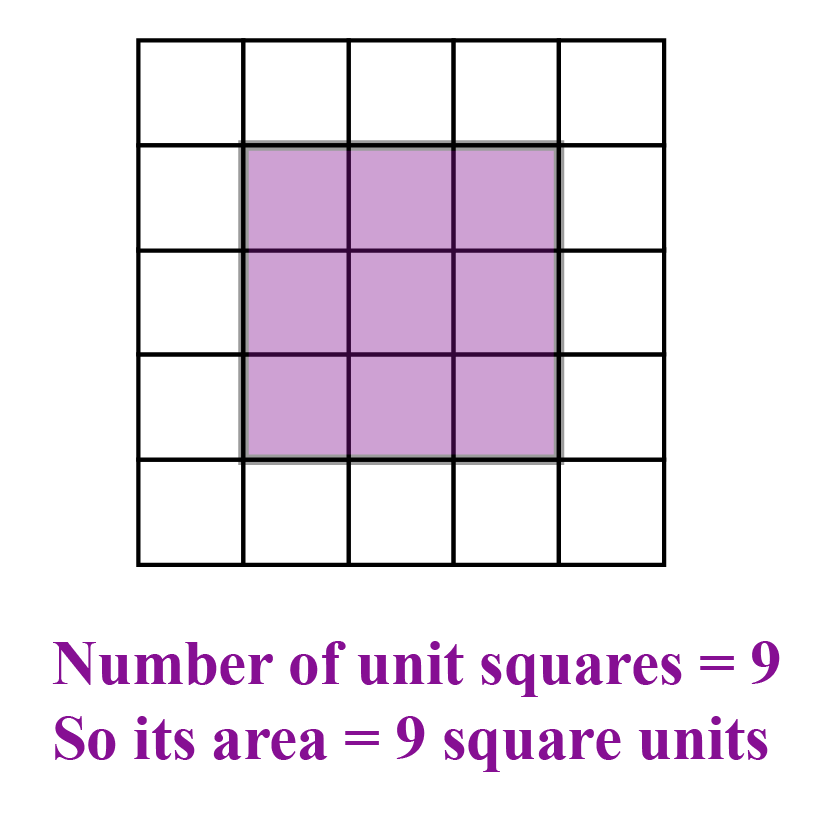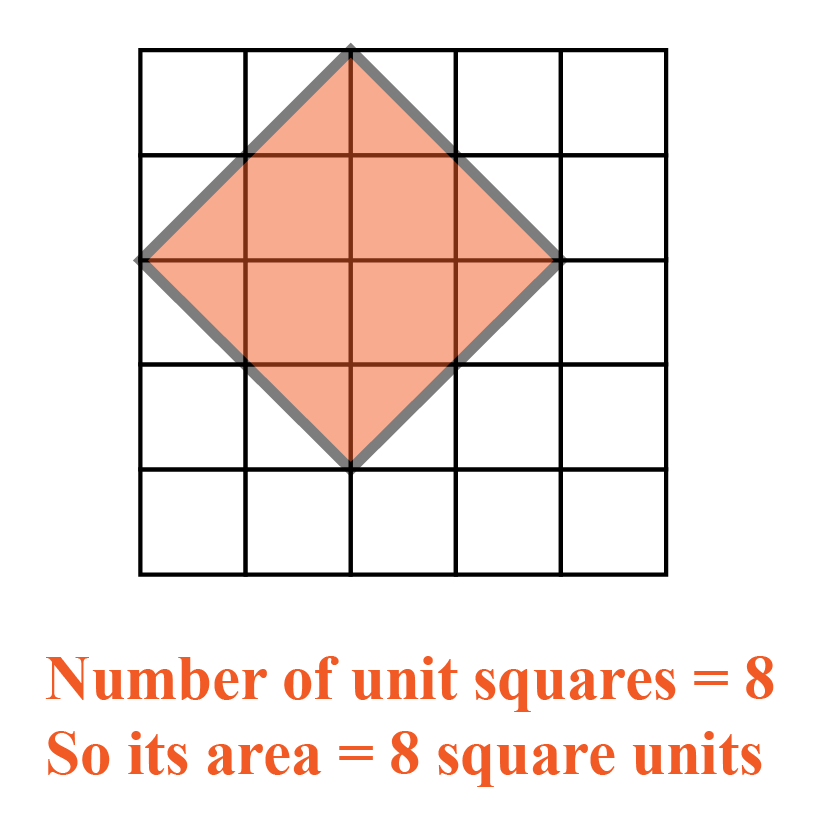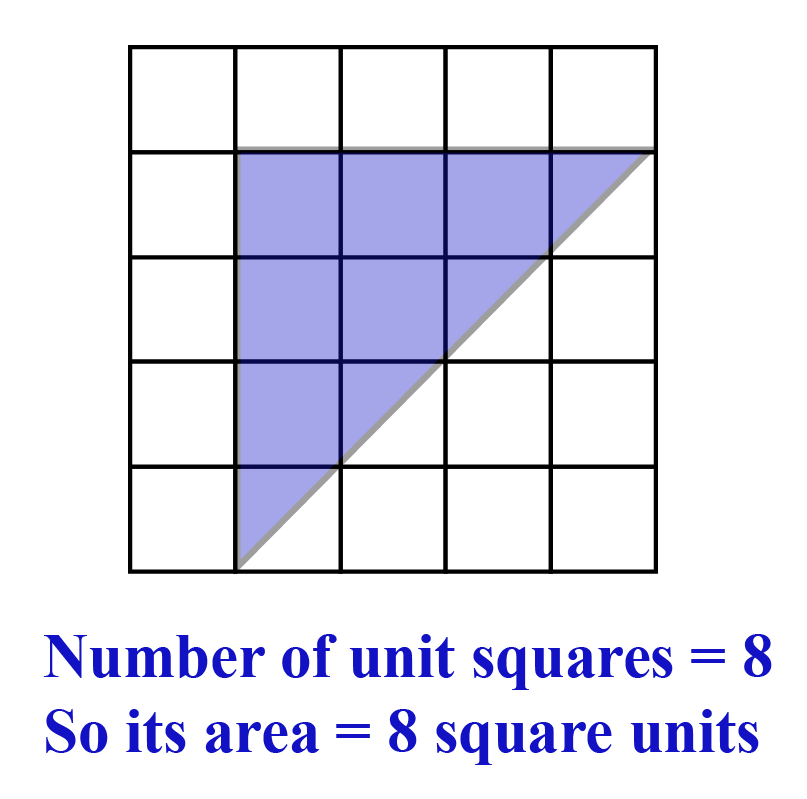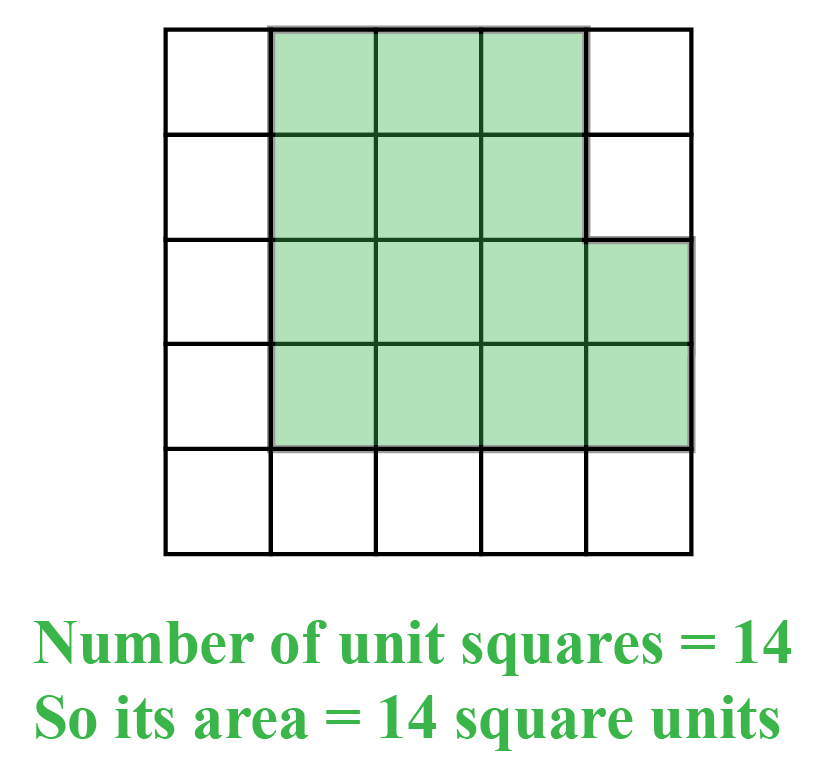We will find the area of a rectangle in a similar way.

## Definition of Area of a Rectangle

As discussed above, the area of a rectangle is the number of unit squares that can fit into it.

For example, let us consider a rectangle of length 4 cm and breadth 3 cm.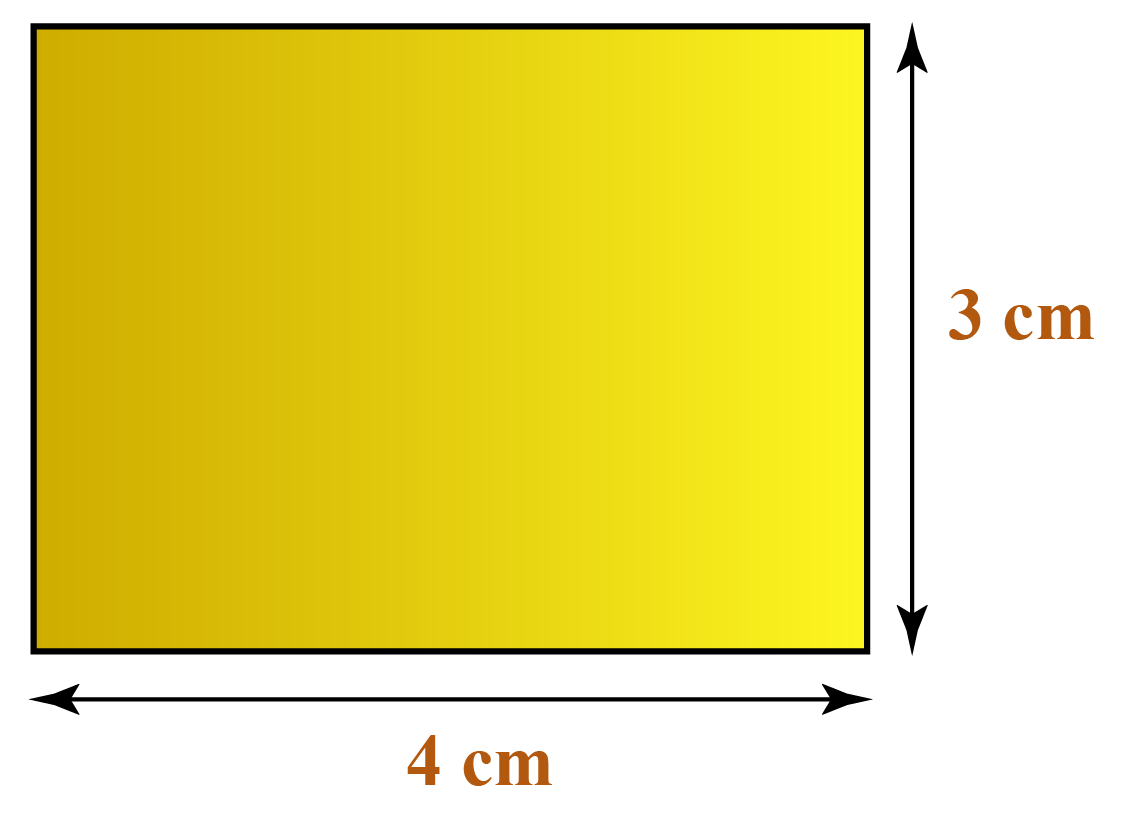Let us draw unit squares inside the rectangle.

Each unit square is a square of length 1 cm.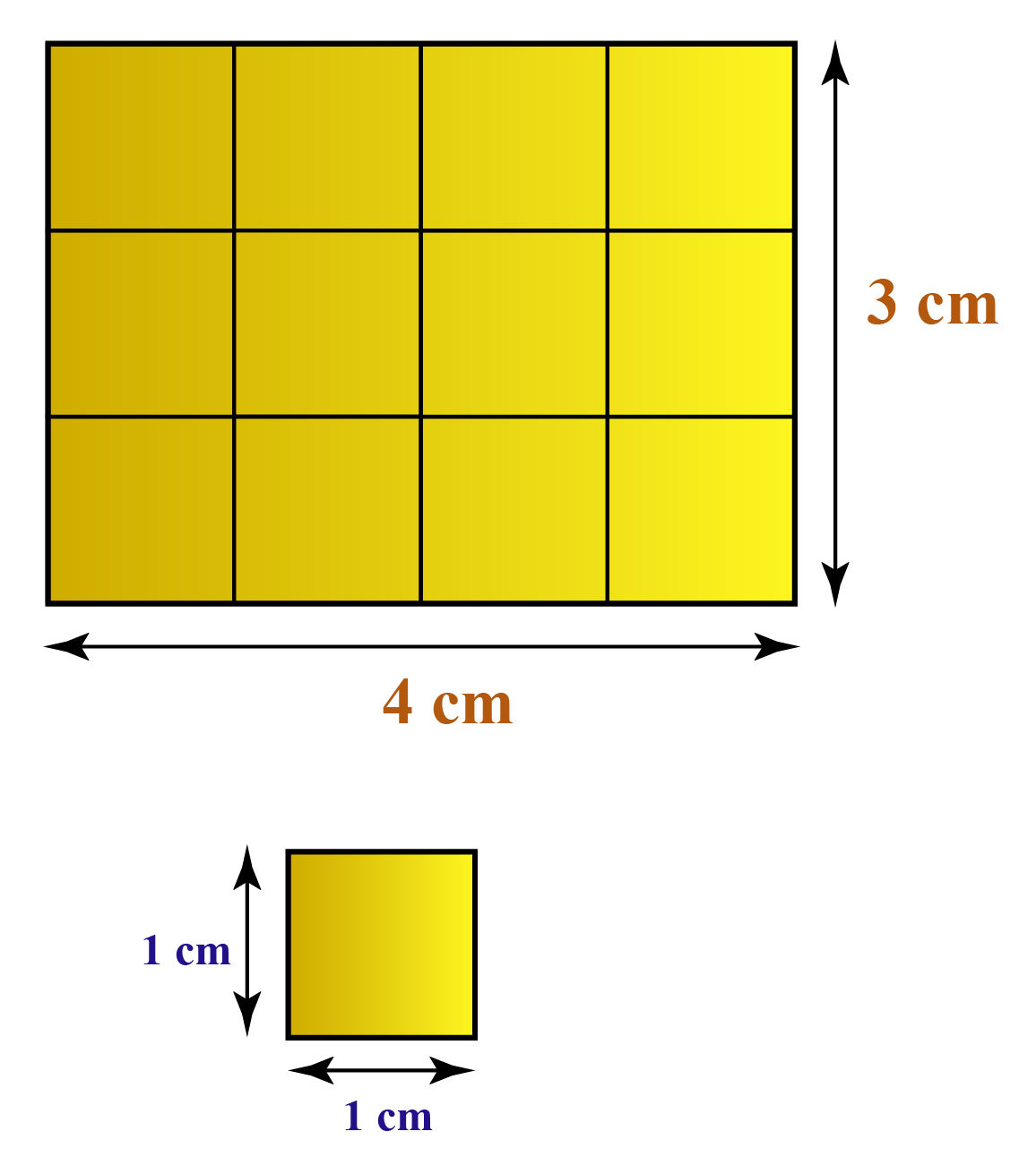Now, count the number of unit squares in the above figure.

How many squares can you observe?

There are 12 squares in total.

We have already learnt that area is measured in square units.

Since the unit of this rectangle is "centimeters", the area is measured in square centimeters which can also be written as $$\text{cm}^2$$.

Thus,

Area of the above rectangle = $${12\text{ cm}^2}$$

You can observe that as we change the length and breadth, the area of the rectangle changes. We can find the area of a rectangle using the following illustration.

## Area of a Rectangle Formula

In the above example, the area of the rectangle whose length is 4 cm and breadth is 3 cm is $$12\text{ cm}^2$$.

We have, $$4 \times 3 = 12$$

The area of a rectangle is obtained by multiplying its length and breadth.

Thus, the formula for the area, $$A$$ of a rectangle whose length and breadth are $$l$$ and $$b$$ respectively is the product $${l \times b}$$.

 $$A=l \times b$$Challenging Questions
1. Find the area of the following figure.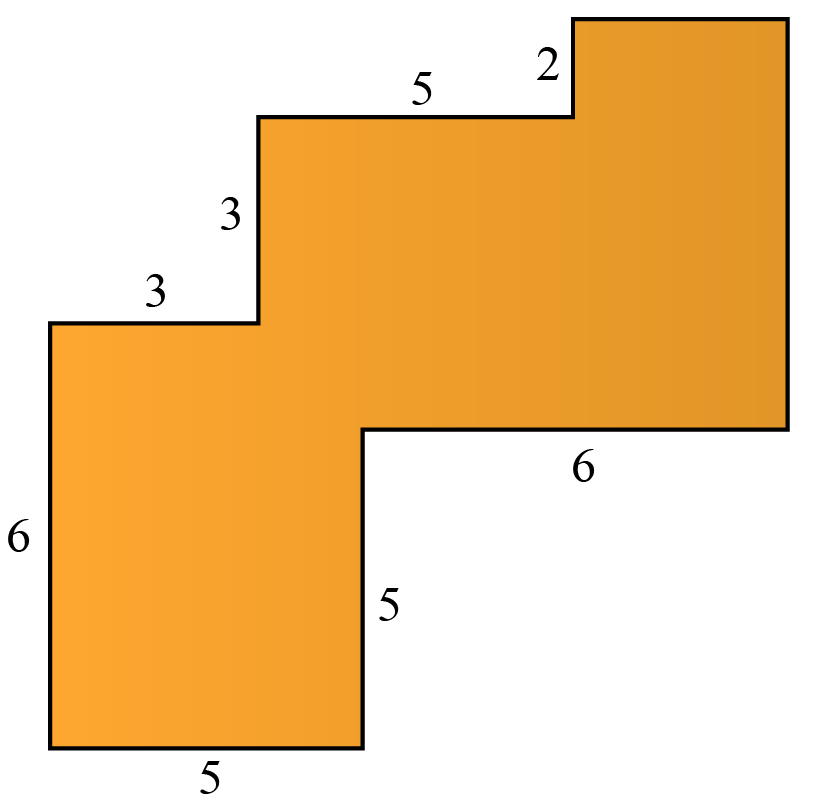CLUEless in Math? Check out how CUEMATH Teachers will explain Area of rectangle to your kid using interactive simulations & worksheets so they never have to memorise anything in Math again!

Explore Cuemath Live, Interactive & Personalised Online Classes to make your kid a Math Expert. Book a FREE trial class today!

## Practice Questions

Here are a few activities for you to practice. Select/Type your answer and click the "Check Answer" button to see the result.

IMO (International Maths Olympiad) is a competitive exam in Mathematics conducted annually for school students. It encourages children to develop their math solving skills from a competition perspective.

## 1. What does the area of a rectangle mean?

The area of a rectangle is the number of unit squares that can fit into it.

The formula for the area 'A' of a rectangle whose length and breadth are 'l' and 'b' respectively is the product l $$\times$$ b

## 2. What is the formula of the area of a rectangle?

The formula for the area 'A' of a rectangle whose length and breadth are 'l' and 'b' respectively is the product l $$\times$$ b

## 3. Define the area of a rectangle.

The area of a rectangle is the number of unit squares that can fit into it.

More Important Topics
Numbers
Algebra
Geometry
Measurement
Money
Data
Trigonometry
Calculus